# Free space in the garden

The grandfather's free space in the garden was in the shape of a rectangular triangle with 5 meters and 12 meters in length. He decided to divide it into two parts and the height of the hypotenuse. For the smaller part creates a rock garden, for the larger sows grass. How many square meters has a larger part?

Result

S =  25.562 m2

#### Solution:

$a=5 \ \text{m} \ \\ b=12 \ \text{m} \ \\ \ \\ c=\sqrt{ a^2+b^2 }=\sqrt{ 5^2+12^2 }=13 \ \\ \ \\ S_{1}=\dfrac{ a \cdot \ b }{ 2 }=\dfrac{ 5 \cdot \ 12 }{ 2 }=30 \ \text{m}^2 \ \\ S_{1}=\dfrac{ c \cdot \ v }{ 2 } \ \\ \ \\ v=\dfrac{ 2 \cdot \ S_{1} }{ c }=\dfrac{ 2 \cdot \ 30 }{ 13 } \doteq \dfrac{ 60 }{ 13 } \doteq 4.6154 \ \text{m} \ \\ \ \\ c c_{1}=a^2 \ \\ c c_{2}=b^2 \ \\ \ \\ c_{1}=\dfrac{ a^2 }{ c }=\dfrac{ 5^2 }{ 13 } \doteq \dfrac{ 25 }{ 13 } \doteq 1.9231 \ \text{m} \ \\ c_{2}=\dfrac{ b^2 }{ c }=\dfrac{ 12^2 }{ 13 } \doteq \dfrac{ 144 }{ 13 } \doteq 11.0769 \ \text{m} \ \\ \ \\ S=\dfrac{ c_{2} \cdot \ v }{ 2 }=\dfrac{ 11.0769 \cdot \ 4.6154 }{ 2 } \doteq \dfrac{ 4320 }{ 169 } \doteq 25.5621 \doteq 25.562 \ \text{m}^2$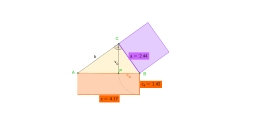Our examples were largely sent or created by pupils and students themselves. Therefore, we would be pleased if you could send us any errors you found, spelling mistakes, or rephasing the example. Thank you!

Leave us a comment of this math problem and its solution (i.e. if it is still somewhat unclear...):Be the first to comment!Tips to related online calculators
Pythagorean theorem is the base for the right triangle calculator.

## Next similar math problems:The quadrilateral pyramid has a rectangular base of 24 cm x 3.2dm and a body height of 0.4m. Calculate its volume and surface area.
2. Hexagonal pyramidCalculate the surface area of a regular hexagonal pyramid with a base inscribed in a circle with a radius of 8 cm and a height of 20 cm.
3. Hexa pyramidThe base of the regular pyramid is a hexagon, which can be described by a circle with a radius of 1 m. Find the volume of the pyramid 2.5 m high.
4. Triangular prism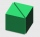Calculate the surface of a triangular prism with the base of an equilateral triangle with a side length of 7.5 cm and a corresponding height of 6.5 cm. Prism height is 15cm.
5. Octagonal pyramidFind the volume of a regular octagonal pyramid with height v = 100 and the angle of the side edge with the plane of the base is α = 60°.
6. Tetrahedral pyramidDetermine the surface of a regular tetrahedral pyramid when its volume is V = 120 and the angle of the sidewall with the base plane is α = 42° 30´.
7. Triangular prism,The regular triangular prism, whose edges are identical, has a surface of 2514 cm ^ 2 (square). Find the volume of this body in cm3 (l).In a regular quadrilateral pyramid, the side edge is e = 7 dm and the diagonal of the base is 50 cm. Calculate the pyramid shell area.
9. Squares above sidesTwo squares are constructed on two sides of the ABC triangle. The square area above the BC side is 25 cm2. The height vc to the side AB is 3 cm long. The heel P of height vc divides the AB side in a 2: 1 ratio. The AC side is longer than the BC side. Calc
10. Diagonal intersectisosceles trapezoid ABCD with length bases | AB | = 6 cm, CD | = 4 cm is divided into 4 triangles by the diagonals intersecting at point S. How much of the area of the trapezoid are ABS and CDS triangles?
11. Playground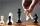On the special playground, there are 81 square sectors, each with a side of 5 m. How many players can fit on the playground if each player needs a 75 m2 area to play?
12. Two bodiesThe rectangle with dimensions 8 cm and 4 cm is rotated 360º first around the longer side to form the first body. Then, we similarly rotate the rectangle around the shorter side b to form a second body. Determine the ratio of surfaces of the first and seco
13. Two 2D shapes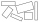Decide which shapes have more area:   (a) a square of 8cm side; or   (b) two rectangles with sides 5cm and 15cm? Write result as 1 or 2 (rectangles)
14. Squares ratioThe first square has a side length of a = 6 cm. The second square has a circumference of 6 dm. Calculate the proportions of the perimeters and the proportions of the contents of these squares? (Write the ratio in the basic form). (Perimeter = 4 * a, conte
15. Cross five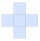The figure on the picture is composed of the same squares and has a content of 45cm². What's his perimeter?
16. Perimeter of the circleCalculate the perimeter of the circle in dm, whose radius equals the side of the square containing 0.49 dm2?
17. Uboid volumeCalculate the cuboid volume if the walls are 30cm², 35cm², 42cm²